Download Presentation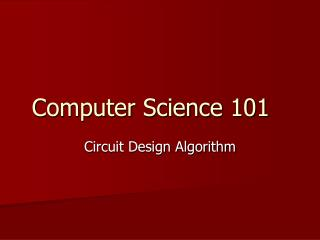Computer Science 101

# Computer Science 101 - PowerPoint PPT Presentation

Computer Science 101 . Circuit Design Algorithm. Circuit Design - The Problem. The problem is to design a circuit that accomplishes a specified task. What does this mean? What kind of task? We would like to specify The number of inputs to the circuit. The number of outputs.I am the owner, or an agent authorized to act on behalf of the owner, of the copyrighted work described.
Download Presentation## Computer Science 101

An Image/Link below is provided (as is) to download presentation

Download Policy: Content on the Website is provided to you AS IS for your information and personal use and may not be sold / licensed / shared on other websites without getting consent from its author.While downloading, if for some reason you are not able to download a presentation, the publisher may have deleted the file from their server.

- - - - - - - - - - - - - - - - - - - - - - - - - - E N D - - - - - - - - - - - - - - - - - - - - - - - - - -
Presentation Transcript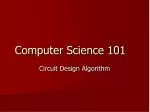### Computer Science 101

Circuit Design Algorithm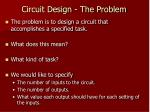Circuit Design - The Problem
• The problem is to design a circuit that accomplishes a specified task.
• What does this mean?
• What kind of task?
• We would like to specify
• The number of inputs to the circuit.
• The number of outputs.
• What value each output should have for each setting of the inputs.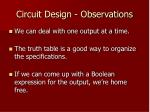Circuit Design - Observations
• We can deal with one output at a time.
• The truth table is a good way to organize the specifications.
• If we can come up with a Boolean expression for the output, we’re home free.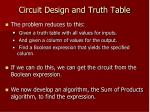Circuit Design and Truth Table
• The problem reduces to this:
• Given a truth table with all values for inputs.
• And given a column of values for the output.
• Find a Boolean expression that yields the specified column.
• If we can do this, we can get the circuit from the Boolean expression.
• We now develop an algorithm, the Sum of Products algorithm, to find the expression.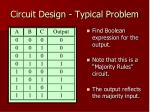Circuit Design - Typical Problem
• Find Boolean expression for the output.
• Note that this is a “Majority Rules” circuit.
• The output reflects the majority input.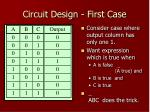Circuit Design - First Case
• Consider case where output column has only one 1.
• Want expression which is true when
• A is false _ (A true) and
• B is true and
• C is true
• _ ABC does the trick.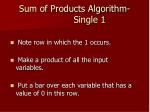Sum of Products Algorithm- Single 1
• Note row in which the 1 occurs.
• Make a product of all the input variables.
• Put a bar over each variable that has a value of 0 in this row.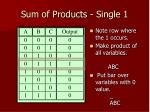Sum of Products - Single 1
• Note row where the 1 occurs.
• Make product of all variables. ABC
• Put bar over variables with 0 value. _ ABC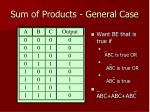Sum of Products - General Case
• Want BE that is true if
• _ ABC is true OR
• _ ABC is true OR
• _ ABC is true
• _ _ _ ABC+ABC+ABC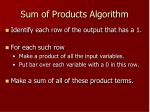Sum of Products Algorithm
• Identify each row of the output that has a 1.
• For each such row
• Make a product of all the input variables.
• Put bar over each variable with a 0 in this row.
• Make a sum of all of these product terms.Sum of Products - Example
• Problem: Design a logic circuit with
• 3 input lines, 1 output line
• Anytime we change the value of any one of the inputs, the output value changes.
• Think of a single light bulb controlled by three light switches that work independently.
• Note: Once we set the output for one set of inputs, the rest is determined.
• We’ll start with all inputs 0 giving output 0.Example continued
• 1’s in output give _ _ _ _ABC + ABC _ _+ ABC + ABCA

B

C

_ _ _ _ _ _

ABC+ABC+ABC+ABC

Boolean Expression

Circuit for the example